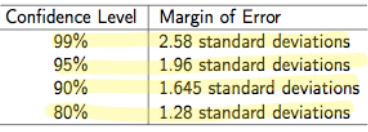Class Notes (1,100,000)
US (480,000)
UCLA (10,000)
STATS (200)
Lecture 13

# STATS 13 Lecture Notes - Lecture 13: Central Limit Theorem, Standard Deviation, Interval Estimation

Department
Statistics
Course Code
STATS 13
Professor
Tsiang, Mike
Lecture
13

This preview shows half of the first page. to view the full 3 pages of the document.Section 3.2 Continued!
!
Conﬁdence intervals!
Deﬁnition!
a conﬁdence interval is an interval or range of plausible values for the population parameter!
conﬁdence level -- level of conﬁdence in this interval!
radius of the conﬁdence interval is called the margin of error!
the conﬁdence level tells us how often out interval estimation method is successful at capturing the true
population parameter, not how often a single observed conﬁdence interval is successful!
!
Simulating!
the true or hypothesized proportion π !
sample size n!
number of repetitions (1000) !
conﬁdence level!
resulting conﬁdence intervals in green contain π!
resulting conﬁdence interval in red do not contain π!
!
Conﬁdence level and Margin of Error!
can change conﬁdence level by changing margin of error!
increasing margin of error increase conﬁdence level!
decreasing margin of error decreases conﬁdence level!
more precise interval = smaller range over population proportion!
a smaller margin of error is helpful but more likely to be wrong-- less conﬁdence!
want a margin of error that will produce an interval that is informative and useful with a good conﬁdence level!
!
selecting a margin of error!
when we have a large enough random sample, the central limit theorem tells us that the sample proportion will be
within about two standard deviations of the population proportion with probability .95!
by choosing two standard deviations as the margin of error, we cover the population proportion in about 95% of
our samples (2SD method)!
p
̂ ± 2SD(p
̂) is a conﬁdence interval for π with an approximate 95% conﬁdence level. !
approximate 95% conﬁdence interval for π !
approximate 68% conﬁdence interval for π is p
̂ ± 1SD(p
̂)!
approximate 95% conﬁdence interval for π is p
̂ ± 2SD(p
̂)!
approximate 99.7% conﬁdence interval for π is p
̂ ± 3SD(p
̂)!
conﬁdence interval for π constructs using theory based approach is also called one sample z interval !
!
Finding balance!
decreasing margin of error from 2 to 1 standard deviation decreases conﬁdence by 27 percentage points (95% to
68%)!
increasing the margin of error from 2 to 3 standard deviations increases conﬁdence by 4.7 percentage points
(95% to 99.7%)!
95% conﬁdence intervals are so common!
two standard deviations usually gives a good balance between high conﬁdence and reasonable margins of
error!
!
Commonly used margins of error!
deﬁnition!
for a given conﬁdence level, margin of error is calculated by!
margin of error = z*SD!
multiplier z* is the number of standard deviations to include in the margin or error!
when z* =1 the conﬁdence level is 68%!
!
!
!
!
!
!